# PMean: Sample size for comparing two diagnostic tests

I had a client come in with a sample size question. It involved the comparison of two diagnostic tests to a gold standard. There a couple of different ways to attack the problem.

The researcher said that he could get about 100 patients, which sounds good at first blush, but it gets a bit tricky. The number of people who would have the disease, as measured by the gold standard, would be about 15% of the sample. So your estimate of sensitivity for either of the two diagnostic tests would have a denominator of 15, which is a bit small. Specificity would be much better off, because the denominator would be 85.

There’s a simple rule of thumb that can help here. When you are testing two groups on a binary outcome measure, you need about 25 to 50 events in each group in order to have adequate precision and power. Here, an event would be the number of patients who test positive or possibly the number who test negative on a particular diagnostic test, both within the group that has the disease by the gold standard and within the group that does not have the disease.

Now this is just a rule of thumb and no one got put in jail for violating a rule of thumb. But we can see a problem here right away. It is very hard to accumulate 25 to 50 positive or negative tests in the sample of 15 patients who have the disease. You might be okay, though, for the sample of 85 patients who do not have the disease, according to the gold standard.

If the sensitivity were 0.8, then a 95% confidence interval based on a denominator of 15 would have a half-width of plus/minus 0.21.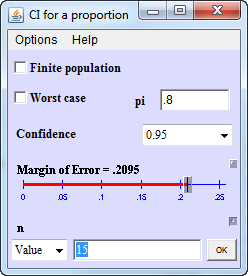That seems much too wide. If the sensitivity were 0.6, the 95% confidence interval would have a width of plus or minus 0.26, which again seems much too wide.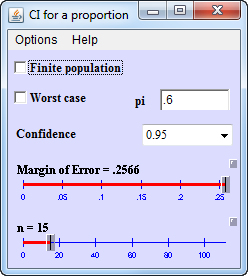Specificity, with a denominator of 85, has very good precision. The half width at a specificity of 0.6, for example would be plus/minus 0.10.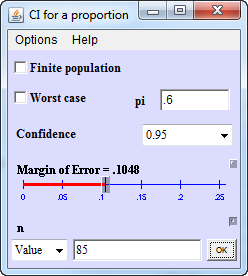Maybe you could live with a study that could only estimate specificity with a reasonable amount of precision. I was not thrilled with these results, though, and neither was my client.

The screenshots, by the way, come from Russ Lenth’s Java applets for power and sample size.

What about comparing the two sensitivities to one another? That might be a bit better, because you are running the both diagnostic tests on the same set of patients. That would seem to improve precision, but for a discrete outcome it is not so clear. What you want to do is test the hypothesis that the sensitivity is better for one diagnostic test versus the other. This would use McNemar’s test. I have a webpage and a newsletter article about McNemar’s test if you want a bit of background first.

How do you justify the sample size for McNemar’s test. It turns out that it depends on the sensitivity of the two tests and the degree to which they tend to agree (e.g., how often, when the first test is positive, does the second test also turn out to be positive). It helps to write out some possible scenarios.

Suppose the first diagnostic test has poor sensitivity (0.6) and the second has better sensitivity (0.8). That means that 9 patients and 12 patients with the disease would test positive under each of the two diagnostic tests, respectively.

The crosstabulation would look like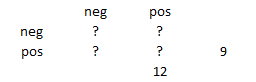Now you have to consider how many of the observations are going to appear on the diagonal and how many are going to appear off the diagonal. You can use a bit of trial and error here. Try to put any value in the lower left corner of the crosstabulation. It has to be smaller than either 9 or 12, but it can’t be too small. Try 7.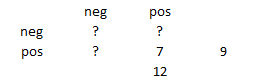You fill in the remaining cells so that the totals add up properly. Here’s what you see.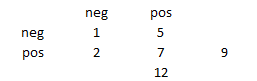You could do this also for 8 instead of 7 or 6 instead of 7. If you tried 5, though, you’d end up with a cell with a negative count.

Now the power of McNemar’s test is dependent on the number of off-diagonal values. The values on the diagonal of the crosstabulation are ignored. In the crosstabulation above, the number of off-diagonal values is 7. You want to test the hypothesis that the probability is 1/2 for either of the two off-diagonal cells. It doesn’t matter which one if you are using a two-sided test. For a reasonable shift in sensitivity from 0.6 to 0.8, the off-diagonal probability would be 5/7 or 0.71. The power of a test that the hypothesized proportion is 0.5, when the proportion is actually 0.71 is very poor.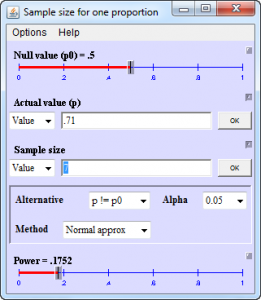This is another of Dr. Lenth’s java applets.

You’d need to examine some of the other possibilities before you called it quits, but it is pretty apparent, either by looking at the width of the confidence intervals for sensitivity or by looking at the power of McNemar’s test for testing the difference in sensitivity between the two diagnostic tests that a total sample of 100 is likely to be too small.

If you could recruit a population where a greater proportion of patients would have the disease, as measured by the gold standard, you might be a bit better off. Perhaps not enough, though. A better solution, if the budget allows it, is to recruit more patients. You might want to strive for 400, which would give you 60 patients who have the disease, according to the gold standard.

This is just an informal set of sample size justifications, and if the client comes back with assurance that a larger sample size is in the cards, then I would run a more formal sample size justification. Still, it is interesting that the informal rule of thumb, the confidence interval calculation, and the power calculation for McNemar’s test all indicate that a total sample size of 100 is inadequate.